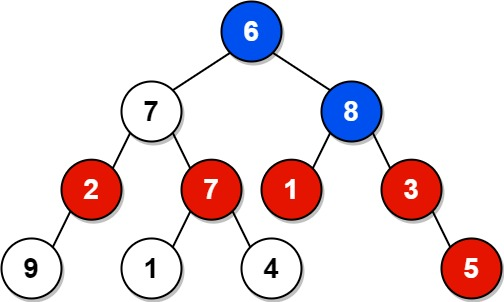# Sum of Nodes with Even-Valued Grandparent

Given the root of a binary tree, return the sum of nodes with an even-valued grandparent. If there are no nodes with an even-valued grandparent, return 0.

grandparent of a node is the parent of its parent if it exists.

Example 1:```Input: root = [6,7,8,2,7,1,3,9,null,1,4,null,null,null,5]
Output: 18
Explanation: Here Node 2 have even valued grandparent Node 6(Marked in Blue).
Likewise all the red nodes are the nodes with even-value grandparent.
Total sum of all the red nodes = 18
```

Example 2:```Input: root = 
Output: 0
Explanation: No grandparent for Node 1 so output is 0```

### Recursive Approach:

For each node, we have to check if its grandparent has an even value.

We can use the Depth First Search approach. How to find the grandparent of the given node? The current node’s parent is a grandparent for its children. Check the below example

```
int compute(Node root, Node parent, Node grandparent)

{

// Current root is passed as Parent and Current root's parent is passed as grandparent
int leftSum = compute(root.left ,root ,parent);
int rightSum = compute(root.right, root, parent);

}

```

If the grandparent of the current node is even-valued, add the value of this node to the total sum. To find the total sum of nodes with even valued grandparent, add the left subtree sum and right subtree sum. In the below example only 2 and 7 have a grandparent. Both of them have even value grandparent 6, so add both to the total sum.### Program:

```class Solution {
public int sumEvenGrandparent(TreeNode root) {

//Tree root doesn't have parent or grandparent so passing it as null
return compute(root,null,null);

}
int compute(TreeNode root, TreeNode parent, TreeNode grandparent)
{
//base condition
if(root == null)
{
return 0;
}

int sum = 0;
int leftSum = compute(root.left, root, parent); // left subtree sum
int rightSum = compute(root.right, root, parent); // right subtree sum
sum += leftSum;
sum += rightSum;

//If the grandparent of the current node is even-valued, add the value of this node to the total sum.
if(grandparent!= null && grandparent.val %2 == 0)
{
sum += root.val;
}
return sum;

}
}

```

### You Might Also Like…

C program to find Height of Binary Tree | Amazon

C program to create a Binary Search Tree | Part1### Sree Hari Sanjeev

The founder of Wisdom Overflow. Software Developer at Zoho Corporation.